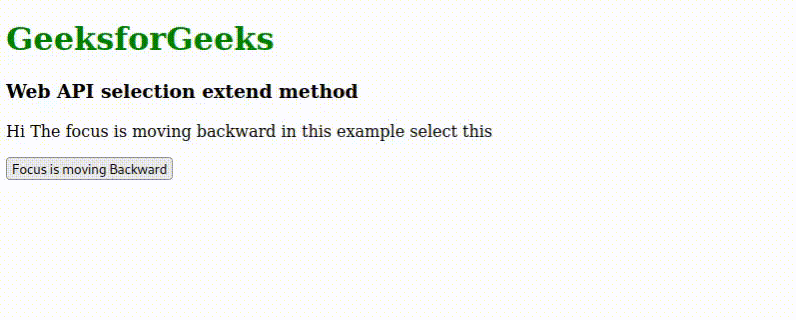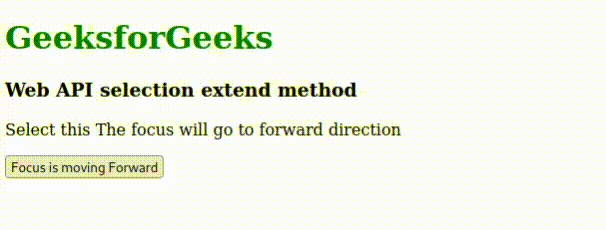Open in App
Not now

# Web API Selection.extend() Method

• Last Updated : 10 Jan, 2023

The Web Selection API gives developers the ability to recognize the screen regions that the user has now selected and to use code to initiate user selections.

The extend() method moves the focus of the selection of a specific point. This method does not work on anchor elements. The focus will start from the anchor of the element, regardless of the direction.

Syntax:

`extend(node, offset)`

Parameters:

• node: This is the node within the focus that will be moved
• offset: The offset position within the node where the focus will be moved to. This is the optional parameter. This parameter value by default is 0

Return value: undefined

Example 1: Below the example, The focus is moving backward not forward. This example shows moving the focus from one node to the second node.

## HTML

 ` ` `<``html``> ` ` `  `<``body``> ` `    ``<``h1` `style``=``"color:green"``>GeeksforGeeks ` `    ``<``h3``> Web API selection extend method ` `    ``<``p``> Hi <``span` `id``=``"spanID"``>  ` `        ``The focus is moving backward in this example ` `        `` select this ` `    `` ` `    ``<``button` `type``=``"button"` `onclick``=``"clickHandler()"``> ` `        ``Click Here!!! ` `    `` ` `    ``<``script``> ` `        ``let node = document.getElementById('spanID') ` ` `  `        ``function clickHandler() { ` `            ``let selection = window.getSelection(); ` `            ``let res = selection.extend(node);             ` `        ``} ` `    `` ` ` ` ` `

Output:Web API Selection.extend() Method

Example 2: Below the code, The focus will be moving forward not backward. Let’s see the example.

## HTML

 ` ` `<``html``> ` ` `  `<``body``> ` `    ``<``h1` `style``=``"color:green"``>GeeksforGeeks ` `    ``<``h3``> Web API selection extend method ` `    ``<``p` `>Select this  ` `        ``<``span` `id``=``"spanID"``>  ` `            ``The focus will go to forward direction ` `        `` ` `    `` ` `    ``<``button` `type``=``"button"` `onclick``=``"clickHandler()"``> ` `        ``Focus is moving Forward ` `    `` ` `    ``<``script``> ` `        ``let node = document.getElementById('spanID') ` ` `  `        ``function clickHandler() { ` `            ``let selection = window.getSelection(); ` `            ``let res = selection.extend(node,1);             ` `        ``} ` `    `` ` ` ` ` `

Output:Web API Selection.extend() Method

Supported Browsers:

• Chrome
• Edge
• Firefox
• Opera
• Safari

My Personal Notes arrow_drop_up
Related Articles